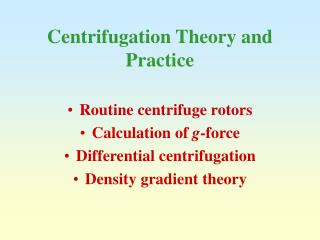DownloadDownload PresentationCentrifugation Theory and Practice

# Centrifugation Theory and Practice

Download Presentation## Centrifugation Theory and Practice

- - - - - - - - - - - - - - - - - - - - - - - - - - - E N D - - - - - - - - - - - - - - - - - - - - - - - - - - -
##### Presentation Transcript

1. Centrifugation Theory and Practice • Routine centrifuge rotors • Calculation of g-force • Differential centrifugation • Density gradient theory

2. axis of rotation Swinging-bucket g At rest Spinning g Fixed-angle Centrifuge rotors

3. axis of rotation rmax rav rmin a rmin rmin rav rav rmax rmax b c Sedimentation path length Geometry of rotors

4. k’-factor of rotors • The k’-factor is a measure of the time taken for a particle to sediment through a sucrose gradient • The most efficient rotors which operate at a high RCF and have a low sedimentation path length therefore have the lowest k’-factors • The centrifugation times (t) and k’-factors for two different rotors (1 and 2) are related by:

5. Calculation of RCF and Q RCF = Relative Centrifugal Force (g-force) Q = rpm; r = radius in cm

6. RCF in swinging-bucket and fixed-angle rotors at 40,000 rpm • Beckman SW41 swinging-bucket (13 ml) • gmin = 119,850g; gav = 196,770g; • gmax = 273,690g • Beckman 70.1Ti fixed-angle rotor (13 ml) • gmin = 72,450g; gav = 109,120g; • gmax = 146,680g

7. v = velocity of sedimentation d = diameter of particle l = density of liquid p = density of particle  = viscosity of liquid g = centrifugal force Velocity of sedimentation of a particle

8. Differential centrifugation • Density of liquid is uniform • Density of liquid << Density of particles • Viscosity of the liquid is low • Consequence: Rate of particle sedimentation depends mainly on its size and the applied g-force.

9. Size of major cell organelles • Nucleus 4-12 m • Plasma membrane sheets 3-20 m • Golgi tubules 1-2 m • Mitochondria 0.4-2.5 m • Lysosomes/peroxisomes 0.4-0.8 m • Microsomal vesicles 0.05-0.3m

10. Decant supernatant 1000g/10 min 3000g/10 min etc. Differential centrifugation of a tissue homogenate (I)

11. Differential centrifugation of a tissue homogenate (II) • Homogenate – 1000g for 10 min • Supernatant from 1 – 3000g for 10 min • Supernatant from 2 – 15,000g for 15 min • Supernatant from 3 – 100,000g for 45 min • Pellet 1 – nuclear • Pellet 2 – “heavy” mitochondrial • Pellet 3 – “light” mitochondrial • Pellet 4 – microsomal

12. Differential centrifugation (III)Expected content of pellets • 1000g pellet: nuclei, plasma membrane sheets • 3000g pellet: large mitochondria, Golgi tubules • 15,000g pellet: small mitochondria, lysosomes, peroxisomes • 100,000g pellet: microsomes

13. Differential centrifugation (IV) • Poor resolution and recovery because of: • Particle size heterogeneity • Particles starting out at rmin have furthest to travel but initially experience lowest RCF • Smaller particles close to rmax have only a short distance to travel and experience the highest RCF

14. Swinging-bucket rotor: Long sedimentation path length gmax >>> gmin Fixed-angle rotor: Shorter sedimentation path length gmax > gmin Differential centrifugation (V)

15. Differential centrifugation (VI) • Rate of sedimentation can be modulated by particle density • Nuclei have an unusually rapid sedimentation rate because of their size AND high density • Golgi tubules do not sediment at 3000g, in spite of their size: they have an unusually low sedimentation rate because of their very low density: (p - l) becomes rate limiting.

16. Density Barrier Discontinuous Continuous Density gradient centrifugation

17. Least dense Most dense How does a gradient separate different particles?

18. Predictions from equation (I) When p > l : v is +ve When p = l : v is 0

19. Predictions from equation (II) When p < l : v is -ve

20. Summary of previous slides • A particle will sediment through a solution if particle density > solution density • If particle density < solution density, particle will float through solution • When particle density = solution density the particle stop sedimenting or floating

21. 1 2 3 4 5 Buoyant density banding Equilibrium density bandingIsopycnic banding

22. 1 2 3 3 Formats for separation of particles accordingto their density When density of particle < density of liquid V is -ve

23. Density Barrier Discontinuous Continuous I II Resolution of density gradients